I.2.7

2/8/2021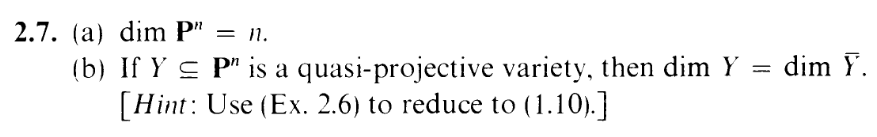HI. Since this is supposed to be a daily thing. I finally wrote a messy bash script (using this sample file) to help me generate these posts. Pls no laff. "L0l0l0l You fucking n00b. Instead of 'basename \$PWD' you should be using '\${PWD##*/}' in order to avoid creating a subprocess and save 0.0000000000001 precious seconds." Yeah, yeah, whatever, fucko. And yes, because the make4ht output has line breaks at very awkward spots, I used some pretty nasty sed commands. I had to learn about BRANCHING, YO.

ANYWAY, let's start the exercise. For part (a), just use yesterday's mess:

 n + 1 = dim S(Pn) = dim Pn + 1
Subtracting 1 on both sides finishes it off.

That was easy. Hey, the hint says to use yesterday's mess for part (b) too!
1.10 is this guy: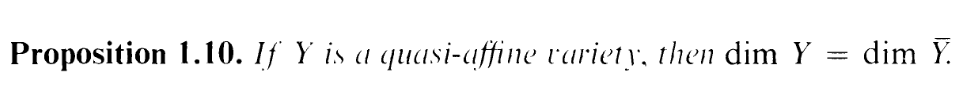(So, it turns out this is another "projectivization of affine" thing)
So how do we hook up 2.6 and 1.10?
Well, Y being a quasi-projective variety means its an open subset of some projective variety. Actually, by 1.6, Y is precisely that variety (or at least the smallest such variety). Now, the bar notation for closure is going to be a little too ambiguous in this exercise, so let's move everything to the cl notation, and we'll be using this property a lot:

"If A is a subspace of X containing S, then the closure of S computed in A is equal to the intersection of A and the closure of S computed in X: clAS = A clXS"

I.e. Let's call X = Y , so we can instead write X = clPnY . And note that clX(Y ) = clPn(Y ) X = X X = X.

. (So our goal in the new notation is to show dim X = dim Y )

Okay. Now let's use the homeomorphism from last time ϕ : X U0 Y 0. We saw last time that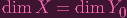(1)

So what happens to the closure of Y when intersected with U0? Well:

 clX∩U0(Y ∩ U0) = clX(Y ∩ U0) ∩ (X ∩ U0) = X ∩ X ∩ U0 1.6 again: since Y and U0 are both open in X = X ∩ U0

And since ϕ is a homeomorphism, closures commute:
 clY 0(ϕ(Y ∩ U0)) = ϕ(clX∩U0(Y ∩ U0)) = ϕ(X ∩ U0) = Y 0

I.e. in "bar" notation: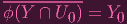(2)

And hence, by 1.10:

 dim Y 0 = dim ϕ(Y ∩ U0) = dim Y ∩ U0 (ϕ is a homeomorphism)
combining this with (1)...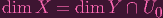(3)

Holy shit this is getting messy. Let's just apply exercise 1.10a to finish it off.

 dim X = dim Y ∩ U0 ≤ dim Y 1.10a
and also by 1.10a, dim Y dim X. DONE.

(ACTUALLY, I just realized I could have just used 1.6 again to say dim Y = dim Y U0. WHATEVS)

Oh, and since I just realized that neocities allows you to upload txt files, I guess I might as well start linking the corresponding tex files for each post. Here's today's one. ALSO, yes, I need to figure out how to not make the equation descriptions jut out of my post. fffuuuuuuuggggg.